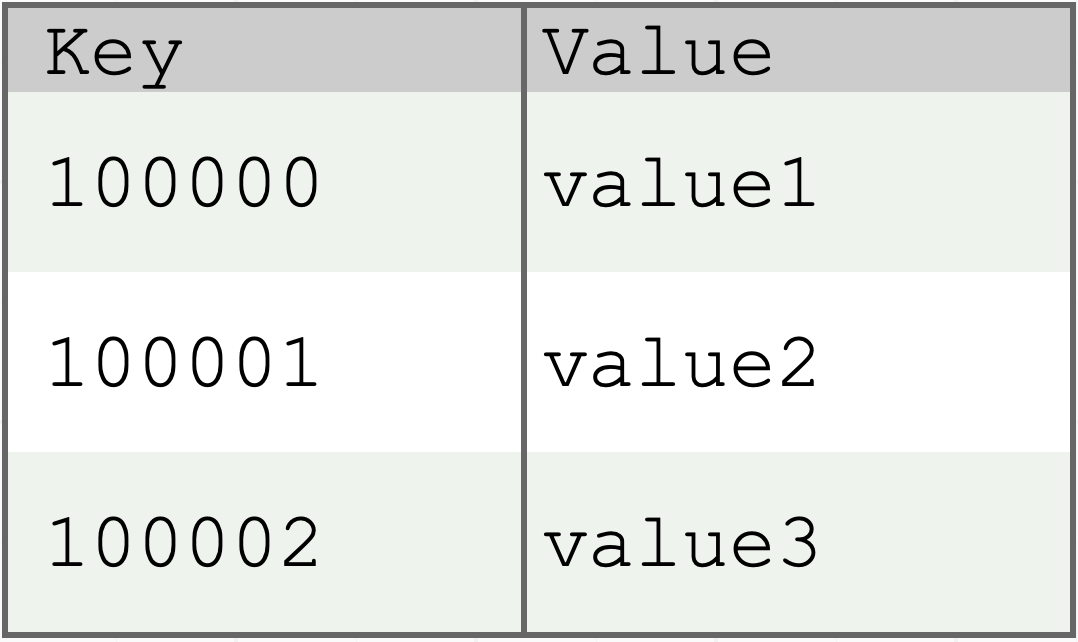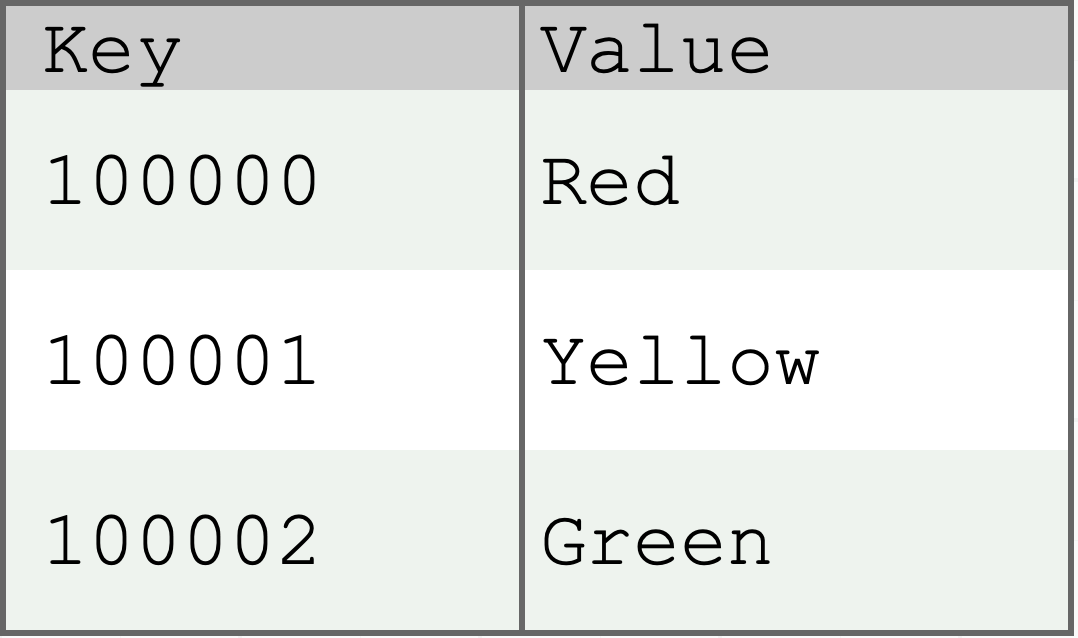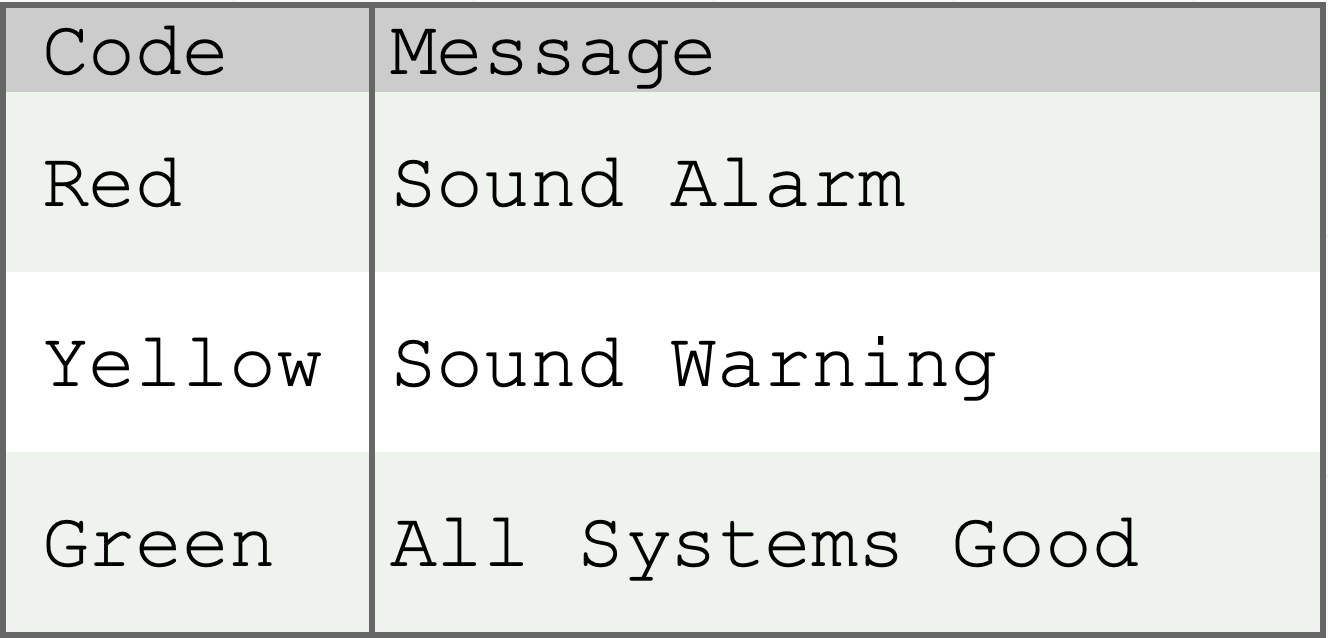# Description

The Test Data Mapping Design Pattern provides different techniques for mapping/pairing synthetically generated test data and actual production data, so that testing may occur without exposing actual production data in the testing environment.

## Definition of a Map

In order to understand Test Data Mapping as a Design Pattern, it is first necessary to understand what a Map is as a concrete concept as it concerns the mapping of data in general. A Map is a data structure that stores a set of Key/Value pairs of data where the given Key identifies and references a given Value.  Within a Map, each Key must be unique and may be associated to only one value.  Within a Map, a Value may be anything (String, Integer, Object, etc.) and a Value does not have to be unique; in essence two or more Keys may map to the same value.

### Abstract Map Example

The data structure below shows an abstract example of a Map having two columns, Key and Value, where keys 100000, 100001 and 100002 are each mapped to an arbitrary value, value1, value2 and value3 respectively.### Concrete Map Example 1

The data structure below shows a concrete example of a Map having two columns, Key and Value, where keys 100000, 100001 and 100002 are each mapped a meaningful value, Red, Yellow and Green respectively.### Concrete Map Example 2

The data structure below shows a concrete example of a Map having two columns, Code and Message (Key and Value), where Code representing keys Red, Yellow and Green are each mapped to a message representing values, Sound Alarm, Sound Warning and All Systems Nominal respectively.## Concrete Test Data Mapping Design Pattern Examples

Now that we have a good understanding of what a Map data structure is and the types of data it is able to contain, we can look at two concrete examples where the Map data structure is utilized in two different concrete test data Scenarios where the Test Data Mapping Design Pattern is utilized to solve the test data challenge, with each test data challenge being solved in a uniquely different way.